📜

• It is just an estimation of the order of magnitude of the length scale in which quantum gravity effects are expected to be important, and quantum gravity is something that we do not have a theory for.
• It's a misnomer to think that it's the fundamental 'pixel' size of the universe or anything like that.
• Physicists say that our current laws of physics break down around this length scale because we don't yet have a single accepted theory of quantum gravity. All our current theories assume that spacetime is continuous (that there is not a minimum length).
• Anyways, a gravitational point singularity is not special in regards to having zero volume. An electron is, as far as we know, also a point particle (in the sense that it has no interior structure), and all experiments to this date have confirmed this with big accuracy. It's of course possible that electrons (or singularities) have a nonzero volume, but to the date no experimental data suggests that this may be the case.
• Alex Klotz, A Hand-Wavy Discussion of the Planck Length
• The Planck length is an extremely small distance constructed from physical constants. There are a lot of misconceptions that generally overstate its physical significance, for example, stating that it’s the inherent pixel size of the universe. The Planck length does have physical significance, and I’ll talk about what it is, and what it isn’t.
• What is the Planck Length?
• Planck units are defined based on physical constants rather than human-scale phenomena. So while the second is originally one-86,400th of a day, the Planck time is based on the speed of light, Newton’s gravitational constant, and Planck’s (reduced) constant, which is twice the angular momentum of an electron. Hypothetically, if we met a group of aliens and wanted to discuss weights and measures, we could use Planck units and they’d know what we are talking about. There is a push towards making our human units based on physical constants, like defining the meter in terms of the speed of light, but at this time the kilogram is still the mass of a brick in France.
• “Natural” units still have a bit of choice regarding their definitions. Convention has chosen Planck’s reduced constant over Planck’s regular constant (they differ by a factor of 2π ), and chosen to use the Coulomb constant instead of dielectric constant or the fundamental charge for electromagnetic units. The latter provides a great example showing that Planck units are not inherently fundamental quantities: the Planck charge is roughly 11.7 times the actual fundamental charge of the universe.
• So what is the Planck length? It is defined as:
• 78a8307425b84c9095e03026513bcb74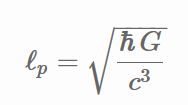• This is how far light can go in a unit of Planck time, because the speed of light is the “Planck speed.” In SI units, this is on the order of 10-35 meters. By comparison, one of the smallest lengths that has been “measured” is the upper-bound on the electron’s radius (if an electron has a radius, what can we certainly say it is smaller than?) is 10-22 meters, about ten-trillion Planck lengths. It is really small. And so far, it is just a unit. The meter is a useful unit for measuring length, but there’s nothing inherently special about it. The Planck length is not useful for measuring any length, but is there anything special about it?
• How is it relevant to physics?
• Basically, the Planck length is the length-scale at which quantum gravity becomes relevant. It is roughly the distance things have to be before you start to consider “hmm I wonder if there’s a chance this whole system randomly forms a black hole.” I did not really understand this until I convinced myself with the following derivation, which was the main inspiration for this article.
• Consider the energy (E) between two charges (let’s say they’re electrons) at some distance r. Doesn’t really matter if they’re attracting or repelling right now.
• 32a4de18460d413baf35771a82bcbe2f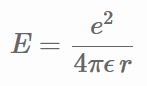• Just to clarify the symbols, e is the fundamental charge, ϵ is the dielectric constant. Now let’s change the units around, using the definition of the fine structure constant α , which is roughly 1/137.
• ef1bc44b9bf1451cb61397209e4a11bd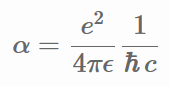• This basically lets us swap out the electromagnetic constants e and ϵ with the more “general” constants ℏ and c. The Coulomb energy now looks like this:
• 5f30fc2c8e824b5a98446812e5c2d3e0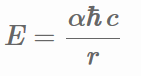• This is where the hand-waving will begin. If a given volume at rest has a certain amount of energy within, it will have a rest mass m=E/c2 . From Newtonian gravity, we can calculate the gravitational energy associated with our charges.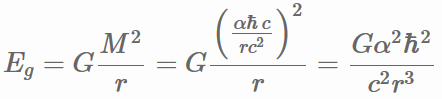• We are neglecting the rest masses of the charges, but those are much smaller than the interaction energy. The question now is: at what distance is the electrostatic energy equal to the gravitational energy it causes? So we solve for r….
• e04ce08647ea4655a930a44a647492ba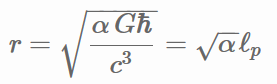• and we find that the radius at which the gravitation of the interaction energy is as important as the interaction energy itself is roughly the Planck length (divided by the 11.7, the square root of 137, but we’ll hand-wave that away for now). This is where it is important: if things are interacting at distances close to the Planck length, you will have to take quantum gravity into account.
• One of the only physical systems where quantum gravity is relevant is the black hole. When calculating the entropy of a black hole, Hawking and Bekenstein found that it was equal to the number of Planck areas (Planck lengths squared) that can fit in the cross-sectional area of a Schwartzschild black hole (or a quarter of its total surface area), in units of the Boltzmann constant. The Hawking temperature of a black hole is one of the only equations where ℏ , c, and G all appear, making it a quantum relativistic gravitational equation. However, the mass of a black hole can be continuous so the number of Planck areas in its surface need not be an integer.
• How is it not relevant to physics?
• There is a misconception that the universe is fundamentally divided into Planck-sized pixels, that nothing can be smaller than the Planck length, that things move through space by progressing one Planck length every Planck time. Judging by the ultimate source, a cursory search of reddit questions, the misconception is fairly common.
• There is nothing in established physics that says this is the case, nothing in general relativity or quantum mechanics pointing to it. I have an idea as to where the misconception might arise, that I can’t really back up but I will state anyway. I think that when people learn that the energy states of electrons in an atom are quantized, and that Planck’s constant is involved, a leap is made towards the pixel fallacy. I remember in my early teens reading about the Planck time in National Geographic, and hearing about Planck’s constant in highschool physics or chemistry, and thinking they were the same.
• As I mentioned earlier, just because units are “natural” it doesn’t mean they are “fundamental,” due to the choice of constants used to define the units. The simplest reason that Planck-pixels don’t make up the universe is special relativity and the idea that all inertial reference frames are equally valid. If there is a rest frame in which the matrix of these Planck-pixels is isotropic, in other frames they would be length contracted in one direction, and moving diagonally with respect to his matrix might impart angle-dependence on how you experience the universe. If an electromagnetic wave with the wavelength of one Planck length were propagating through space, its wavelength could be made even smaller by transforming to a reference frame in which the wavelength is even smaller, so the idea of rest-frame equivalence and a minimal length are inconsistent with one-another.
• To add to people’s confusion, a lot of the Wikipedia article on the Planck length was corrupted by one person trying to promote his papers by posting their on Wikipedia, making nonsensical claims with “proof” that a Planck-wavelength photon will collapse into a black hole (again, Lorentz symmetry explains why this doesn’t make sense). There is a surreal and amusing dialogue trying to get to the bottom of this, that you can still read in the discussion section of the Planck length Wikipedia page.
• There was an analysis recently of gamma ray arrival times from a burst in a distant galaxy. The author considered what effect a discretization of space might have on the travel speed of photons of differing energy (it would no longer necessarily be constant), and found that to explain the observations the length-scale of the discretization must be at least 525 smaller than the Planck-length. I’m not too sure how seriously people in the field take this paper.
• How might it be relevant to physics?
• Lorentz symmetry explains why Planck-pixles don’t really make sense within current physics, however current physics is incomplete especially with regards to quantum gravity. Going beyond established physics, is there more of a roll for the Planck length? I’m a bit out of my element talking about this, so I’ll be brief.
• The closest beyond-standard theory to the Planck-pixel idea is Loop Quantum Gravity and the concept of quantum foam. At least that is what I thought, before John Baez corrected me. One of the features of Loop Quantum Gravity is that for something to have a surface area or a volume, it must have at least a certain quantum value of surface area or volume, but will not necessarily have integer values of that quantum, and the quantum is not exactly the square or cube of the Planck length, although it is of that order.
• Another potential model of quantum gravity is string theory, based on the dynamics of really small strings. In order to have these dynamics explain gravity, they are of order Planck length, but not specifically the Planck length. In fact, the first iteration of string theory was theorized to explain nuclear physics rather than gravity, and the length-scale of the strings was much much larger.
• So to summarize, the Planck length is an important order of magnitude when quantum gravity is being discussed, but it is not the fundamental pixel size of the universe. Thanks to John Baez and Nima Lashkari for answering some questions about quantum gravity.